Back to Contents

# In and Out

We have considered a function (and indeed, a whole program composed of many functions) to take a chunk of data, do some calculations, and then produce a result. This assumption has allowed us to write neat, easily understood programs.

However, some computer programs do not have all data available at the beginning of the program (or even the beginning of a given function). The user might provide new data interactively, or the program might fetch data from the internet, or two or more programs might communicate with one another in real time.

We must learn how to write such programs, whilst understanding the utility of restricting such complications to as small a part of the program as possible – interactivity turns out to be surprisingly hard to reason about, since the result of a function may no longer depend only on its initial argument.

### Writing to the screen

OCaml has a built-in function `print_int` which prints an integer to the screen:

` OCaml`

`# print_int 100;;`
`100- : unit = ()`

What is the type of this function? Well, it is a function, and it takes an integer as its argument. It prints the integer to the screen, and then returns…what? Nothing! OCaml has a special type to represent nothing, called unit. There is exactly one thing of type unit which is written `()` and is called “unit”. So, the function `print_int` has type int unit.

There is another built-in function `print_string` of type string unit to print a string, and another `print_newline` to move to the next line. This function has type unit unit because it requires no substantive argument and produces no useful result. It is only wanted for its “side-effect”.

We can produce several side-effects, one after another, using the `;` symbol. This evaluates the expression on its left hand side, throws away the result (which will normally be unit anyway), and then evaluates the expression to its right hand side, returning the result (which is often unit too). The type of the expression x `;` y is thus the type of y. For example, we can write a function to write to the screen an int × string pair as an integer on one line, followed by a string on another: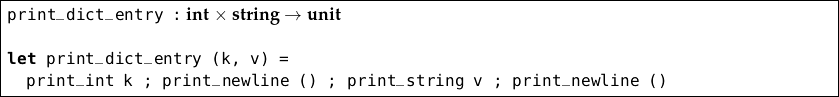Notice we have added a second call to `print_newline`, so that our function can be called several times in a row without intervening calls to `print_newline`. We wrote the function applications all on one line to emphasize that `;` behaves a little like an operator. However, for convenience, we would normally write it like this: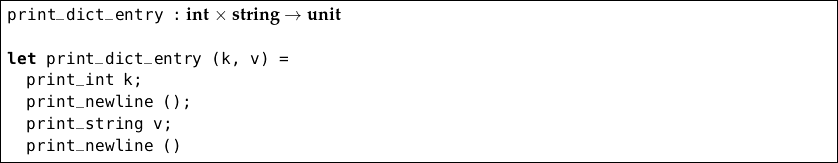This makes it look rather like `;` is used to end each expression, but just remember that `;` is a bit like an operator – notice that there is no `;` after the last `print_newline ()`. Let us see how `print_dict_entry` is used in practice:

` OCaml`

`# print_dict_entry (1, "one");;`
`1`
`one`
`- : unit = ()`

How might we print a whole dictionary (represented as a list of entries) this way? Well, we could write our own function to iterate over all the entries: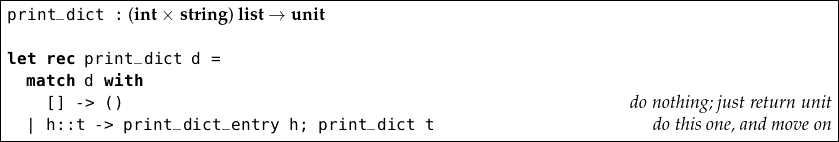Better, we can extract this method into a more general one, for doing an action on each element of a list: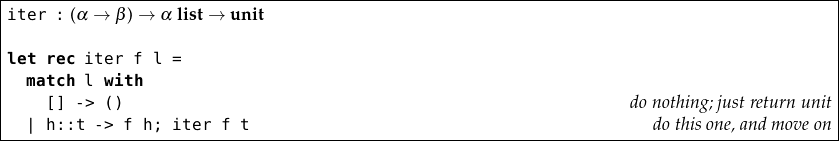Normally β will be unit. Now we can redefine `print_dict` using `iter`: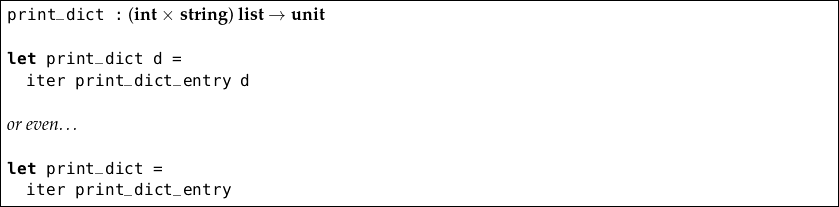For example:

` OCaml`

`# print_dict [(1, "one"); (2, "two"); (3, "three")];;`
`1`
`one`
`2`
`two`
`3`
`three`
`- : unit = ()`

Now we should like to write a function to read a dictionary as an (int × string) list. We will use two built-in OCaml functions. The function `read_int` of type unit int waits for the user to type in an integer and press the Enter key. The integer is then returned. The function `read_line` of type unit string waits for the user to type any string and press the enter key, returning the string.

We want the user to enter a series of keys and values (integers and strings), one per line. They will enter zero for the integer to indicate no more input. Our function will take no argument, and return a dictionary of integers and strings, so its type will be unit (int × string) list.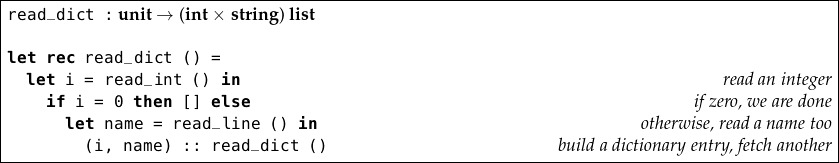We can run this function and type in some suitable values:

` OCaml`

`# read_dict ();;`
`1`
`oak`
`2`
`ash`
`3`
`elm`
`0`
`- : (int * string) list = [(1, "oak"); (2, "ash"); (3, "elm")]`

But there is a problem. What happens if we type in something which is not an integer when an integer is expected?

` OCaml`

`# read_dict ();;`
`1`
`oak`
`ash`
`Exception: Failure "int_of_string".`

We must handle this exception, and ask the user to try again. Here’s a revised function: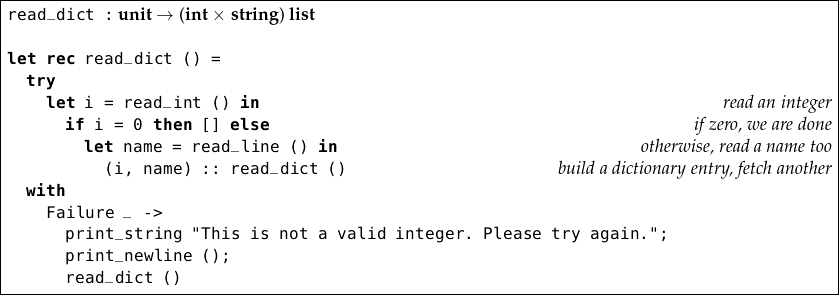Now, typing mistakes can be fixed interactively:

` OCaml`

`# read_dict ();;`
`1`
`oak`
`ash`
`This is not a valid integer. Please try again.`
`2`
`ash`
`3`
`elm`
`0`
`- : (int * string) list = [(1, "oak"); (2, "ash"); (3, "elm")]`

### Using files

It is inconvenient to have to type new data sets in each time, so we will write functions to store a dictionary to a file, and then to read it back out again.

OCaml has some basic functions to help us read and write from places data can be stored, such as files. Places we can read from have type in_channel and places we can write to have type out_channel. Here are functions for writing a dictionary of type (int × string) to a channel: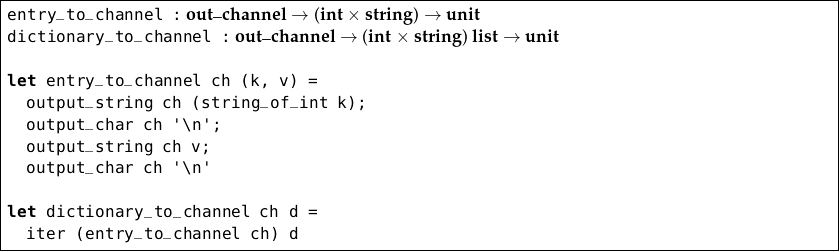We are using the functions `output_string` and `output_char` to write the data in the same format we used to print it to the screen. There is no `output_int` function, so we have used the built-in `string_of_int` function to build a string from the integer. The character `’\n’` is a special one, representing moving to the next line (there is no `output_newline` function).

How do we obtain such a channel? The function `open_out` gives an output channel for filename given as a string. It has type string out_channel. After we have written the contents to the file, we must call `close_out` (which has type out_channel unit) to properly close the file.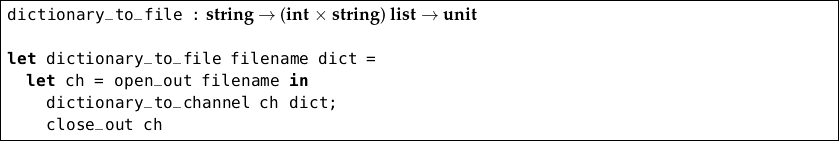After running this function, you should find a file of the chosen name on your computer in the same folder from which you are running OCaml. If you are not sure where the file is being put, consult the documentation for your OCaml implementation, or use a full file path such as `"C:/file.txt"` or `"/home/yourname/file.txt"`, again depending on your system. In the following example, we are reading a dictionary from the user and writing it to file as `file.txt`:

` OCaml`

`# dictionary_to_file "file.txt" (read_dict ());;`
`1`
`oak`
`2`
`ash`
`3`
`elm`
`0`
`- : unit`

Now we have written a file, we can read it back in: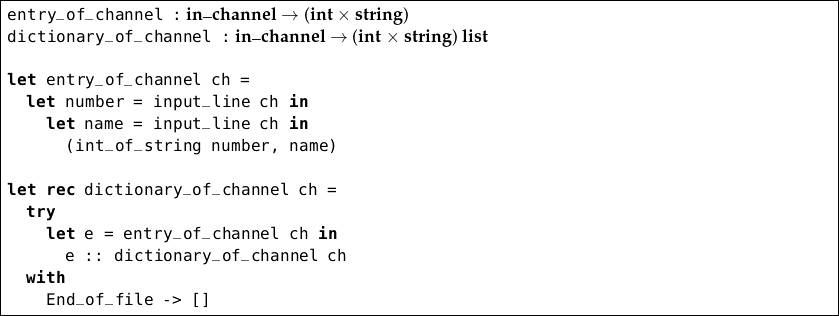We have written a function `entry_of_channel` to read a single integer and string (one element of our dictionary) from an input channel using the built-in functions `input_line` and `int_of_string`, and a function `dictionary_of_channel` to read all of them as a dictionary. It makes use of the built-in exception `End_of_file` to detect when there is no more in the file. Now, we can build the main function to read our dictionary from the file: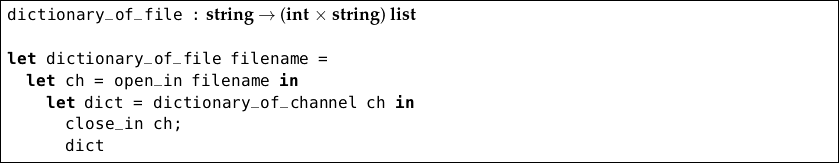The process is the same as for `dictionary_to_file` but we use `open_in` and `close_in` instead of `open_out` and `close_out`.

` OCaml`

`# dictionary_of_file "file.txt";;`
`- : (int * string) list = [(1, "oak"); (2, "ash"); (3, "elm")]`

### Summary of functions

We have introduced the types unit, in_channel, and out_channel, and the exception `End_of_file`. Here are the functions we have used: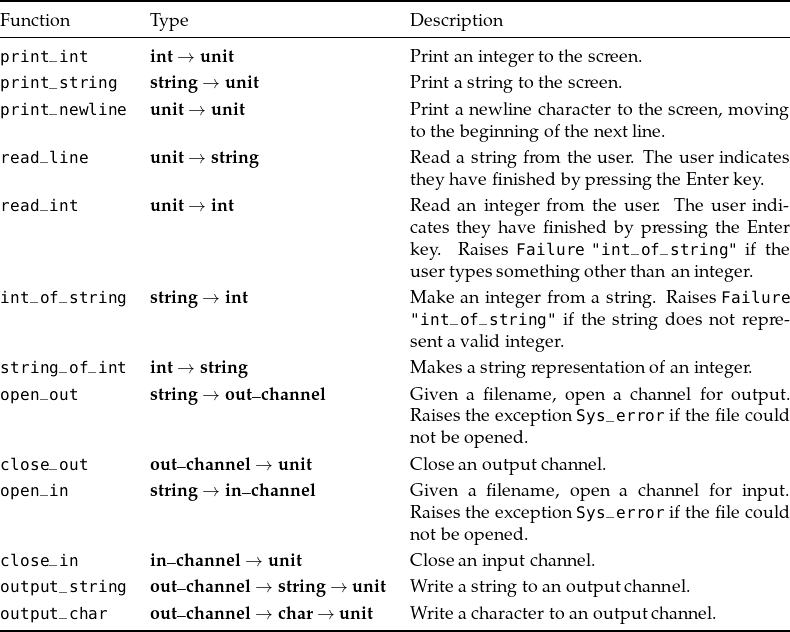## Questions

1. Write a function to print a list of integers to the screen in the same format OCaml uses – i.e. with square brackets and semicolons.

2. Write a function to read three integers from the user, and return them as a tuple. What exceptions could be raised in the process? Handle them appropriately.

3. In our `read_dict` function, we waited for the user to type `0` to indicate no more data. This is clumsy. Implement a new `read_dict` function with a nicer system. Be careful to deal with possible exceptions which may be raised.

4. Write a function which, given a number x, prints the x-times table to a given file name. For example, `table "table.txt" 5` should produce a file `table.txt` containing the following: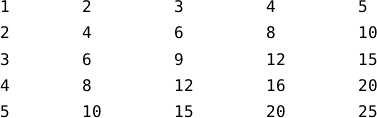Adding the special tabulation character `\t` after each number will line up the columns.

5. Write a function to count the number of lines in a given file.

6. Write a function `copy_file` of type string string unit which copies a file line by line. For example, `copy_file "a.txt" "b.txt"` should produce a file `b.txt` identical to `a.txt`. Make sure you deal with the case where the file `a.txt` cannot be found, or where `b.txt` cannot be created or filled.